强化学习_深度强化学习和强化学习 - CSDN
• 强化学习实战视频培训教程概况：强化学习是当下爆火的机器学习经典模型，系列课程从实例出发，形象解读强化学习如何完整一个实际任务。由基本概念过度到马尔科夫决策过程，通过实例演示如何通过迭代求解来得出来好...深度学习 深度学习框架 深度学习算法 python深度学习 深度强化学习
• 深入浅出地介绍强化学习的概念，算法发展历史，分类，及发展趋势。 强化学习深入浅出完全教程，内容包括强化学习概述、马尔科夫决策过程、基于模型的动态规划方法、蒙特卡罗方法、时间差分方法、Gym环境构建及...强化学习 深度强化学习 强化学习matlab 多智能体强化学习 强化学习路径规划
• DQN（Deep Q-Learning）可谓是深度强化学习（Deep Reinforcement Learning，DRL）的开山之作，是将深度学习与强化学习结合起来从而实现从感知（Perception）到动作（ Action ）的端对端（End-to-end）学习的一种...
转载自：https://blog.csdn.net/u013236946/article/details/72871858

DQN（Deep Q-Learning）可谓是深度强化学习（Deep Reinforcement Learning，DRL）的开山之作，是将深度学习与强化学习结合起来从而实现从感知（Perception）到动作（ Action ）的端对端（End-to-end）学习的一种全新的算法。由DeepMind在NIPS 2013上发表1，后又在Nature 2015上提出改进版本2。

一、DRL

原因：在普通的Q-learning中，当状态和动作空间是离散且维数不高时可使用Q-Table储存每个状态动作对的Q值，而当状态和动作空间是高维连续时，使用Q-Table不现实。

通常做法是把Q-Table的更新问题变成一个函数拟合问题，相近的状态得到相近的输出动作。如下式，通过更新参数 θ

使Q函数逼近最优Q值

Q(s,a;θ)≈Q′(s,a)

而深度神经网络可以自动提取复杂特征，因此，面对高维且连续的状态使用深度神经网络最合适不过了。

DRL是将深度学习（DL）与强化学习（RL）结合，直接从高维原始数据学习控制策略。而DQN是DRL的其中一种算法，它要做的就是将卷积神经网络（CNN）和Q-Learning结合起来，CNN的输入是原始图像数据（作为状态State），输出则是每个动作Action对应的价值评估Value Function（Q值）。

二、DL与RL结合的问题

DL需要大量带标签的样本进行监督学习；RL只有reward返回值，而且伴随着噪声，延迟（过了几十毫秒才返回），稀疏（很多State的reward是0）等问题；
DL的样本独立；RL前后state状态相关；
DL目标分布固定；RL的分布一直变化，比如你玩一个游戏，一个关卡和下一个关卡的状态分布是不同的，所以训练好了前一个关卡，下一个关卡又要重新训练；
过往的研究表明，使用非线性网络表示值函数时出现不稳定等问题。
三、DQN解决问题方法

通过Q-Learning使用reward来构造标签（对应问题1）
通过experience replay（经验池）的方法来解决相关性及非静态分布问题（对应问题2、3）
使用一个CNN（MainNet）产生当前Q值，使用另外一个CNN（Target）产生Target Q值（对应问题4）
1、构造标签

前面提到DQN中的CNN作用是对在高维且连续状态下的Q-Table做函数拟合，而对于函数优化问题，监督学习的一般方法是先确定Loss Function，然后求梯度，使用随机梯度下降等方法更新参数。DQN则基于Q-Learning来确定Loss Function。

Q-Learning
有关RL的基础知识不再啰嗦，直接看Q-Learning的更新公式：

Q∗(s,a)=Q(s,a)+α(r+γmaxa′Q(s′,a′)−Q(s,a))

而DQN的Loss Function为

L(θ)=E[(TargetQ−Q(s,a;θ))2]

其中 θ 是网络参数，目标为

TargetQ=r+γmaxa′Q(s′,a′;θ)

显然Loss Function是基于Q-Learning更新公式的第二项确定的，两个公式意义相同，都是使当前的Q值逼近Target Q值。

接下来，求 L(θ)

关于 θ 的梯度，使用SGD等方法更新网络参数 θ

。

2、经验池（experience replay）

经验池的功能主要是解决相关性及非静态分布问题。具体做法是把每个时间步agent与环境交互得到的转移样本 (st,at,rt,st+1)

储存到回放记忆单元，要训练时就随机拿出一些（minibatch）来训练。（其实就是将游戏的过程打成碎片存储，训练时随机抽取就避免了相关性问题）

3、目标网络

在Nature 2015版本的DQN中提出了这个改进，使用另一个网络（这里称为TargetNet）产生Target Q值。具体地，Q(s,a;θi)

表示当前网络MainNet的输出，用来评估当前状态动作对的值函数；Q(s,a;θ−i) 表示TargetNet的输出，代入上面求 TargetQ

值的公式中得到目标Q值。根据上面的Loss Function更新MainNet的参数，每经过N轮迭代，将MainNet的参数复制给TargetNet。

引入TargetNet后，再一段时间里目标Q值使保持不变的，一定程度降低了当前Q值和目标Q值的相关性，提高了算法稳定性。

四、DQN算法流程

1、网络模型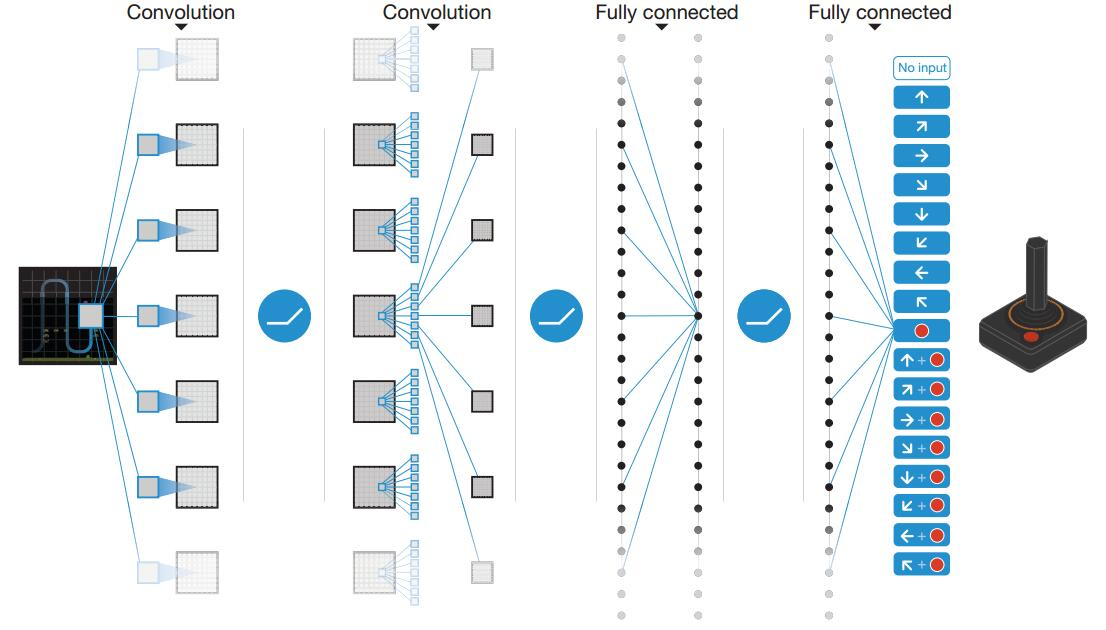输入的是被处理成灰度图的最近4帧 84×84

图像，经过几个卷积层（没有池化层）后接两个全连接层，输出是所有动作的Q值。

2、算法伪代码

NIPS 2013版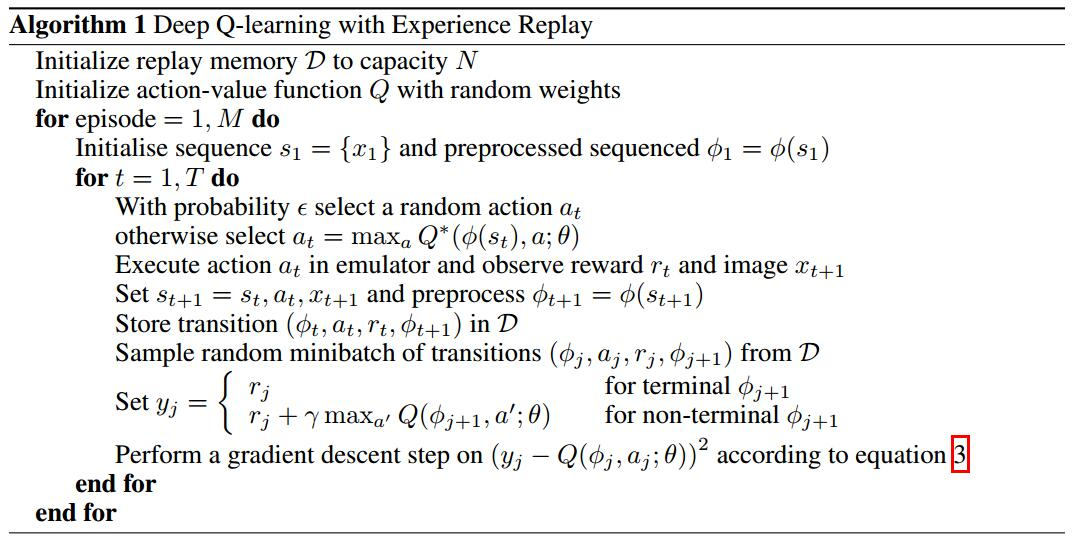Nature 2015版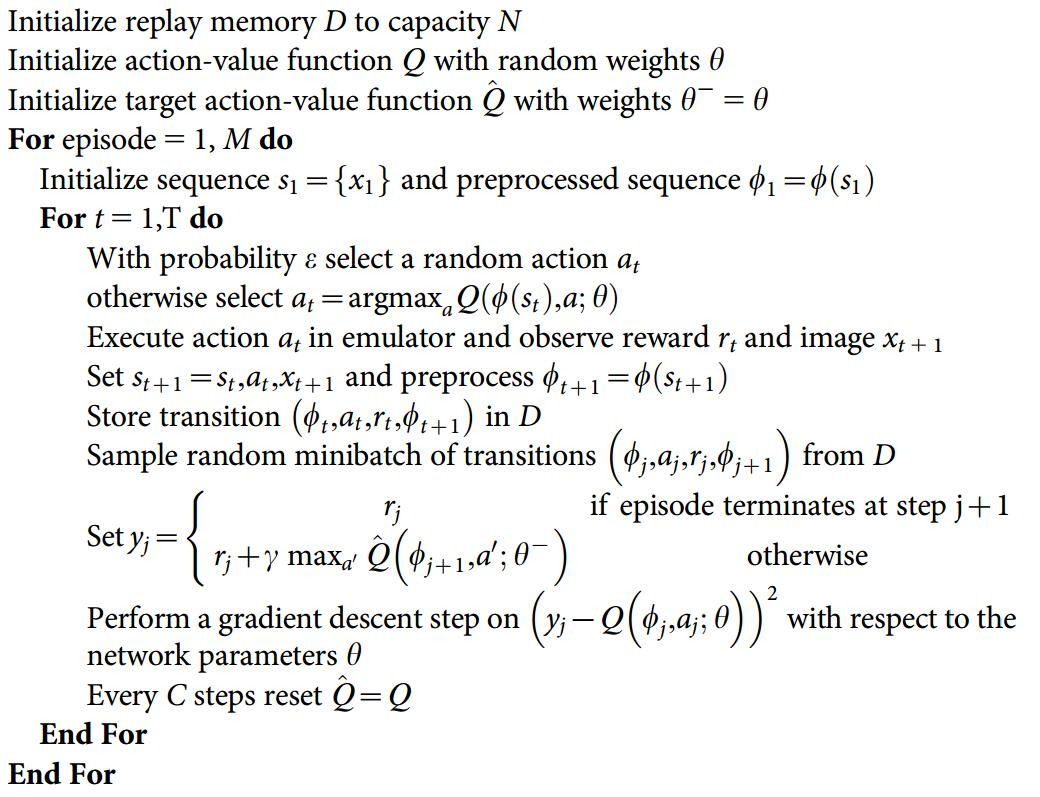2、算法流程图（2015版）

主要流程图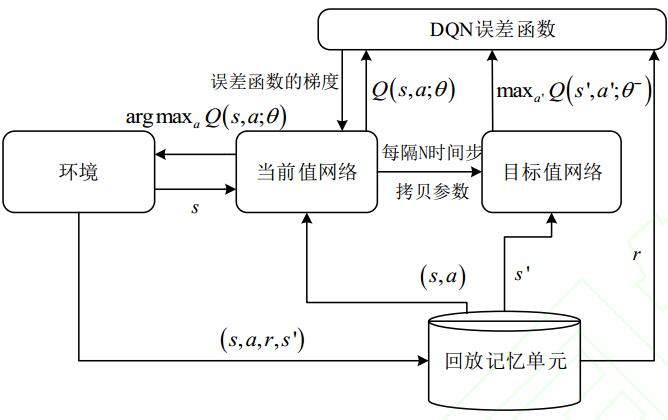Loss Function 的构造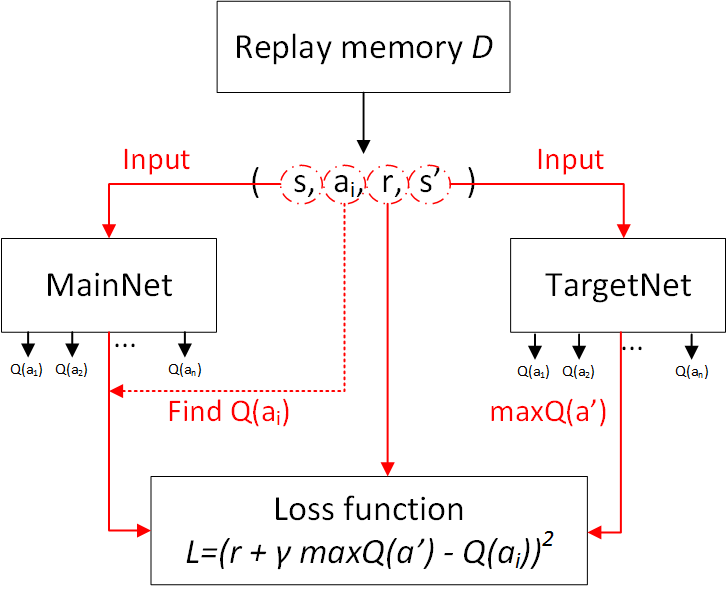五、总结

DQN是第一个将深度学习模型与强化学习结合在一起从而成功地直接从高维的输入学习控制策略。

创新点：

基于Q-Learning构造Loss Function（不算很新，过往使用线性和非线性函数拟合Q-Table时就是这样做）。
通过experience replay（经验池）解决相关性及非静态分布问题；
使用TargetNet解决稳定性问题。
优点：

算法通用性，可玩不同游戏；
End-to-End 训练方式；
可生产大量样本供监督学习。
缺点：

无法应用于连续动作控制；
只能处理只需短时记忆问题，无法处理需长时记忆问题（后续研究提出了使用LSTM等改进方法）；
CNN不一定收敛，需精良调参。

展开全文• 强化学习强化学习介绍 1.定义 强化学习(reinforcement learning)，又称再励学习、评价学习，是一种重要的机器学习方法，在智能控制机器人及分析预测等领域有许多应用。但在传统的机器学习分类中没有...
【强化学习】强化学习介绍

引用morvan老师的教学视频和DeepMind的公开课

学习资料搬砖

David Silver 的 UCL 强化学习课程：http://www0.cs.ucl.ac.uk/staff/d.silver/web/Teaching.html
Sergey Levine 教授的深度强化学习课程：http://rail.eecs.berkeley.edu/deeprlcourse/
OpenAI 的 Spinning Up in Deep RL：https://blog.openai.com/spinning-up-in-deep-rl/
Sutton 和 Barto 的强化学习著作：http://incompleteideas.net/book/the-book-2nd.html
一本有关深度强化学习的书籍草稿：https://arxiv.org/abs/1810.06339
一份深度强化学习资源汇集：https://medium.com/@yuxili/resources-for-deep-reinforcement-learning-a5fdf2dc730f

1.定义
强化学习(reinforcement learning)，又称再励学习、评价学习，是一种重要的机器学习方法，在智能控制机器人及分析预测等领域有许多应用。但在传统的机器学习分类中没有提到过强化学习，而在连接主义学习中，把学习算法分为三种类型，即非监督学习(unsupervised learning)、监督学习(supervised leaning)和强化学习。
强化学习主要包含四个元素：智能体（Agent）、环境状态（environment）、奖励（reward）、动作（action）。
举个例子作为一个决策者（agent）你有两个动作（action）学习、打游戏，你去打游戏学习成绩就会下降，学习成绩就是你的（state）你的父母看到你学习成绩会对你相应的奖励和惩罚（reward）所以经过一定次数的迭代你就会知道什么样的成绩下选择什么样的动作会能够得到最高的奖励的，这就是强化学习的主要思想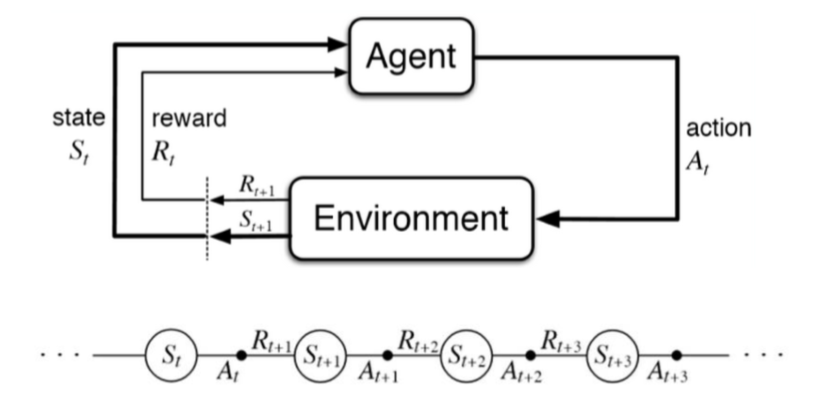2.强化学习算法分类
在模型已知的MDP情况下，已知MDP的状态转移概率和回报函数的动态规划方法有四种：

线性规划方法 利用线性规划求解值函数
Policy Iteration 策略迭代的方式，求解最优策略。
Value Iteration 值迭代为了得到各个状态下采取什么动作能够的得到最大奖励。
广义策略迭代方法  关注点是每一步的最优行动。

3.强化学习主要算法
Q_Learning
强化学习值迭代主要算法，主要通过环境获得当前环境s_t下选取对应动作a获取的r，然后将其构建成一张Q表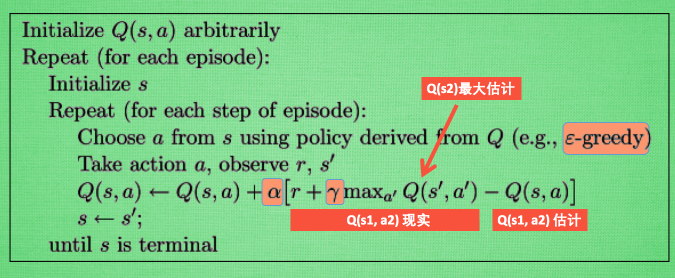Sarsa
Sarsa的计算Q(s1, a2) 现实的时候,  是去掉maxQ的，遵循说道做到原则, 取而代之的是在 s2 上我们实实在在选取的 a2 的 Q 值. 最后像 Q learning 一样, 求出现实和估计的差距 并更新 Q 表里的 Q(s1, a2).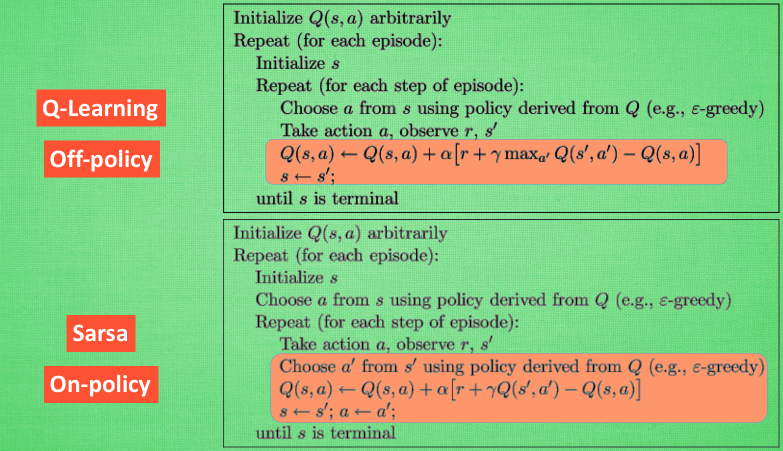Deep Q Network（DQN）
因为DQN采用了神经网络来计算Qvalue所以就可以替换掉Qlearning中的Q-table，根据状态s和动作a直接来计算相应Q值，然后进行值迭代。DQN 在玩游戏上应用广泛适用于状态空间和动作空间过大的场景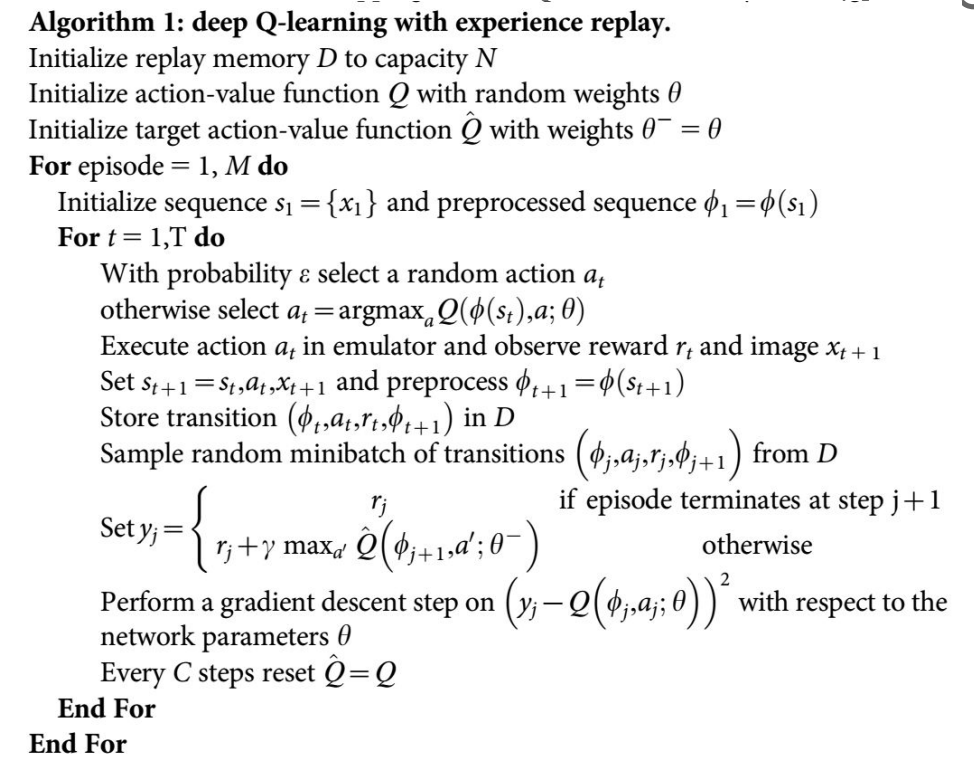Policy Grandient
policy grandient与以往的算法不同，它并非通过计算Q（s，a）值来选择动作，而是直接输出行为，他的好处是可以在一个连续的区间内挑选动作，普通的Qlearning在无穷多动作下计算值是困难的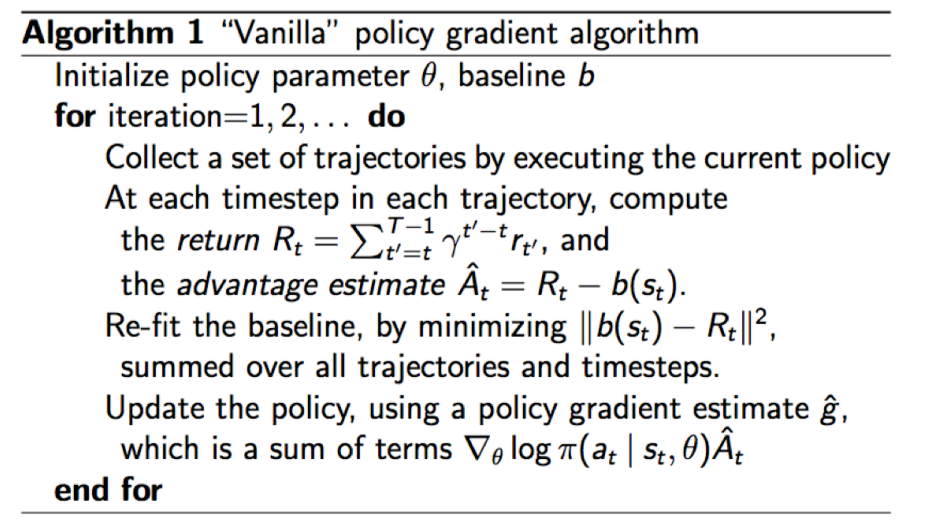Actor Critic
Actor-Critic 的 Actor 是由 Policy Gradients演变而来, 他能在连续动作中选取合适的动作, 而 Q-learning 做这件事会瘫痪.又因为Actor Critic 是根据Q-learning 或者其他的 以值为基础的学习法来的 , 能进行单步更新, 而传统的 Policy Gradients 则是回合更新, 这降低了学习效率.
Critic被用来学习奖惩机制，就是环境和奖励之间的联系，所以可以对actor的动作进行反馈，使得Actor每一步都在更新。同时还可以衍生出可以分布式运行的A3C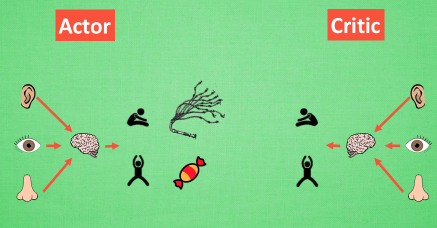将DQN 网络加入进 Actor Critic 系统中, 这种新算法叫做 Deep Deterministic Policy Gradient, 成功的解决的在连续动作预测上的学不到东西问题，DDPG分为两个部分策略 Policy 的神经网络 和基于 价值 Value 的神经网络, 但是为了体现 DQN 的思想, 每种神经网络我们都需要再细分为两个动作估计值网络，和状态估计值网络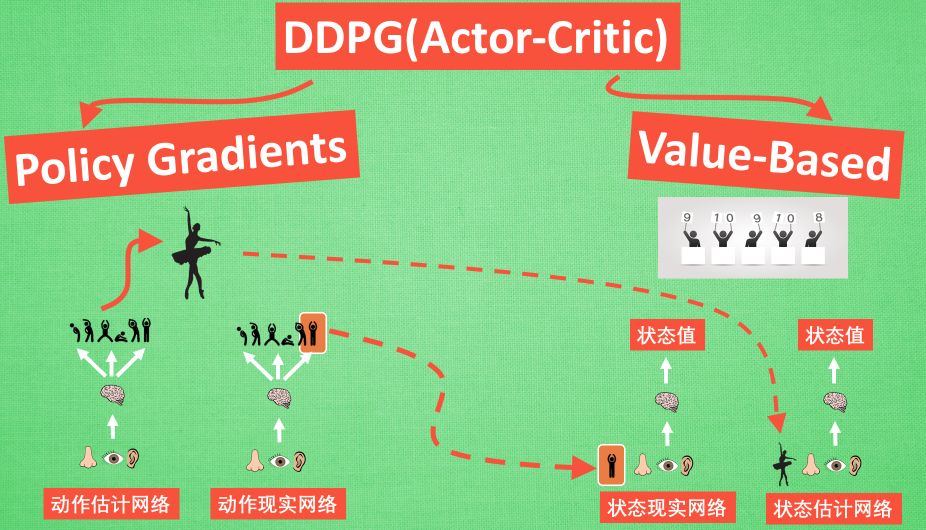这些算法主要来源于morvan老师的教学视频和DeepMind的公开课，算法详细内容与公式推导见接下来的详细讲解


展开全文强化学习
• 强化学习：原理与Python实战》是一本配套TensorFlow 2代码的强化学习教程书，全书完整地介绍了主流的强化学习理论，听众可以了解强化学习基础知识，通过实例感受强化学习的魅力，并了解强化学习前沿进展。强化学习 深度强化学习 强化学习matlab 多智能体强化学习 强化学习路径规划
• Last login: Tue Apr 11 10:44:01 on ttys003 MacBook-Pro:~ baiducdProject/MacBook−Pro:Projectbaidu cd Project/ MacBook-Pro:Project baidu ls baidu caffe kaldi personal
前言

近几年，由于DeepMind成功地将强化学习（reinforcement learning）运用在AlphaGo上，机器首次在复杂任务上取得了超过人类的表现，使得强化学习成为目前机器学习研究的前沿方向之一。强化学习由来已久，Sutton等在1979年就已经开始研究强化学习，1998年出版了强化学习介绍一书，并于2012年发布第二版，本文前几部分内容主要参考该书。

强化学习最早主要用于智能控制领域，比如机器人控制、电梯调度、电信通讯等，如今已经在自动驾驶、NLP、内容推荐和语音交互领域都有相关的应用。2013年底DeepMind发表文章Playing Atari with Deep Reinforcement Learning，首次成功地将深度学习运用到强化学习任务上，通过无监督学习实现从纯图像输入来玩Atari 2600游戏的效果。而后DeepMind逐渐改进算法，使得DQN在Atari几乎一半的游戏中超过人类水平，以至2016年AlphaGo和无人车的出现，人们惊奇地发现人工智能即将颠覆我们的生活，甚至有人评论说传统的深度学习已经可以很好地感知理解了，强化学习可以利用这些感知生成策略，因而可以创造更高的机器智能。

下面是DeepMind使用DQN让机器学习玩Atari 2600游戏的视频。

什么是强化学习

Reinforcement learning is learning what to do—how to map situations to actions—so as to maximize a numerical reward signal.

强化学习研究的是智能体agent与环境之间交互的任务，也就是让agent像人类一样通过试错，不断地学习在不同的环境下做出最优的动作，而不是有监督地直接告诉agent在什么环境下应该做出什么动作。在这里我们需要引入回报（reward）这个概念，回报是执行一个动作或一系列动作后得到的奖励，比如在游戏超级玛丽中，向上跳可以获得一个金币，也就是回报值为1，而不跳时回报就是0。回报又分为立即回报和长期回报，立即回报指的是执行当前动作后能立刻获得的奖励，但很多时候我们执行一个动作后并不能立即得到回报，而是在游戏结束时才能返回一个回报值，这就是长期回报。强化学习唯一的准则就是学习通过一序列的最优动作，获得最大的长期回报。比较有挑战性的是，任一状态下做出的动作不仅影响当前状态的立即回报，而且也会影响到下一个状态，因此也就会影响整个执行过程的回报。

因此，强化学习和监督学习的区别主要有以下两点：

强化学习是试错学习(Trail-and-error)，由于没有直接的指导信息，智能体要以不断与环境进行交互，通过试错的方式来获得最佳策略。
延迟回报，强化学习的指导信息很少，而且往往是在事后（最后一个状态）才给出的，这就导致了一个问题，就是获得正回报或者负回报以后，如何将回报分配给前面的状态。

问题描述与MDP

前面已经提到强化学习是尝试并发现回报最大动作的过程，下面就具体来描述一下这个过程。首先考虑一个问题，一个之前完全没有接触过国际象棋的小白怎样和一个专业棋手对弈。刚开始小白对棋面并没有任何概念，只能随机下，但假设双方每一轮下完后都会得到立即回报，比如吃子回报为1，被吃回报为-1，其他回报为0。可以想象一开始小白会输得很惨，但如果小白很聪明，随着不断地尝试小白不仅理解了下棋的规则，并且知道在什么棋面下做出什么动作可以吃更多的棋子。在这里我们将小白作为我们的智能体agent，棋面就是状态，下棋就是agent根据当前状态做出的动作，每个动作执行完后都会引起状态改变，如果状态的改变只与前一个状态和当前的动作有关，而与之前的状态和动作无关（即满足马尔可夫性），那么整个过程可以用马尔可夫决策过程（Markov Decision Processes）来描述，而Sutton在书中直接将满足马尔可夫性的强化学习任务定义为马尔可夫决策过程，并将状态和动作都是有限空间的MDP定义为有限马尔可夫决策过程（finite MDP）。

下面引入一些定义：马尔可夫决策过程是一个agent与环境交互的过程，因此有一个离散的时间序列，t=0,1,2,3,...$t=0,1,2,3,...$，在每一个时刻t$t$，agent都会接收一个用来表示环境的状态St∈S$S_{t}\in\bf{S}$，其中S$\bf{S}$表示所有可能状态的集合，并且在状态的基础上选择一个动作At∈A(St)$A_{t}\in{\bf{A}}(S_{t})$，其中A(St)${\bf{A}}(S_{t})$表示在状态St$S_{t}$时所有可能采取的动作的集合，在t$t$时刻agent采取一个动作后都会收到一个回报值Rt+1∈R$R_{t+1}\in\bf{R}$，然后接收一个新状态St+1$S_{t+1}$。下图为整个过程的示意图。在任意时刻和状态下，agent都可以选择一个动作，选择的依据就是我们说的策略—即状态到动作的映射π(a∣s)$\pi(a\mid{s})$，而一个使得在任意时刻和状态下的长期回报都是最大的策略是我们最终需要得到的。所谓长期回报我们可以用每个时刻的立即回报来表示：

Gt=Rt+1+Rt+2+Rt+3+...=∑∞k=t+1Rk(1.1)$G_{t}=R_{t+1}+R_{t+2}+R_{t+3}+...=\sum_{k=t+1}^{\infty}R_{k}\tag{1.1}$

但实际上我们一般会用下面更通用的公式来代替：

Gt=Rt+1+γRt+2+γ2Rt+3+...+γT−t−1RT=∑T−t−1k=0γkRt+k+1(1.2)$G_{t}=R_{t+1}+\gamma{R_{t+2}}+\gamma^2{R_{t+3}}+...+\gamma^{T-t-1}{R_{T}}=\sum_{k=0}^{T-t-1}\gamma^{k}R_{t+k+1}\tag{1.2}$

其中γ∈[0,1]$\gamma\in[0,1]$称为回报折扣因子，表明了未来的回报相对于当前回报的重要程度。γ=0$\gamma=0$时，相当于只考虑立即回报不考虑长期回报，γ=1$\gamma=1$时，将长期回报和立即回报看得同等重要。T∈[1,∞]$T\in[1,\infty]$表示完成一次实验过程的总步数，T=∞$T=\infty$和γ=1$\gamma=1$不能同时满足，否则长期回报将无法收敛。特别地，我们将一次有限步数的实验称作一个单独的episodes，也就是经过有限步数后最终会接收一个终止状态，这一类的任务也叫做episodic tasks。下面讨论的强化学习任务都是有限MDP的episodic tasks。

马尔可夫决策过程

一个有限马尔可夫决策过程由一个四元组构成 M=(S,A,P,R)$M=({\bf{S}}, {\bf{A}}, {\bf{P}}, {\bf{R}})$。如上所述，S$\bf{S}$表示状态集空间，A${\bf{A}}$表示动作集空间，P${\bf{P}}$表示状态转移概率矩阵，R${\bf{R}}$表示期望回报值。

在MDP中给定任何一个状态s∈S$s\in\bf{S}$和动作a∈A$a\in\bf{A}$，都会以某个概率转移到下一个状态s′$s^{'}$，这个概率为p(s′∣s,a)=Pr{St+1=s′∣St=s,At=a}∈P$p(s^{'}\mid s, a)={\bf{Pr}}\{S_{t+1}=s^{'}\mid S_{t}=s, A_{t}=a\}\in\bf{P}$，并获得下一个回报的期望值为r(s,a,s′)=E[Rt+1∣St=s,At=a,St+1=s′]∈R$r(s,a,s^{'})={\bf{E}}\left[R_{t+1}\mid{S_{t}=s,A_{t}=a,S_{t+1}=s^{'}}\right]\in\bf{R}$。

值函数及贝尔曼公式

增强学习的最终结果是找到一个环境到动作的映射—即策略π(a∣s)$\pi(a\mid{s})$。如果一个策略只考虑立即回报，那么很可能就会掉入眼前陷阱。比如说有一个岔路口，往左回报是100，往右回报是10，如果策略只考虑立即回报，那肯定是往左，但往左走的下一次回报只有10，而往右走的下一次回报有200，可以看到这个策略并不是最优的策略，此外增强学习又往往有具有延迟回报的特点，在很多情况下的动作并不会产生立即回报，但这一系列动作的累积效果又的确会导致后续回报的产生，因此立即回报并不能说明策略的好坏。在几乎所有的强化学习理论中都会定义值函数来表示给定策略下期望的未来回报，并将值函数作为评估学习效果的指标。

值函数有多种定义，目前常见的是将值函数直接定义为未来回报的期望：

υπ(s)=Eπ[Gt∣St=s]=Eπ[∑∞k=0γkRt+k+1∣St=s](2.1)$\upsilon_{\pi}(s)={\bf{E_{\pi}}}\left[G_{t}\mid{S_{t}=s}\right]={\bf{E_{\pi}}}\left[\sum_{k=0}^{\infty}\gamma^{k}R_{t+k+1}\mid{S_{t}=s}\right]\tag{2.1}$

上面表示的是在某个策略π$\pi$下，当环境处于状态s$s$时未来回报的期望，因此又叫做状态值函数(state-value function for policy)，只跟当前状态有关。同样，我们也可以定义动作值函数(action-value function for policy)，如下：

qπ(s,a)=Eπ[Gt∣St=s,At=a]=Eπ[∑∞k=0γkRt+k+1∣St=s,At=a](2.2)$q_{\pi}(s,a)={\bf{E_{\pi}}}\left[G_{t}\mid{S_{t}=s,A_{t}=a}\right]={\bf{E_{\pi}}}\left[\sum_{k=0}^{\infty}\gamma^{k}R_{t+k+1}\mid{S_{t}=s,A_{t}=a}\right]\tag{2.2}$

动作值函数表示在某个策略π$\pi$下，当环境处于状态s$s$时采取动作a$a$的未来回报的期望。可以看到动作值函数与状态值函数唯一的不同是动作值函数不仅指定了一个初始状态，而且也指定了初始动作，而状态值函数的初始动作是根据策略产生的。由于在MDP中，给定状态s$s$，agent根据策略选择动作a$a$，下个时刻将以概率p(s′∣s,a)$p(s^{'}\mid{s,a})$转移到状态s′$s^{'}$，因此值函数又可以改写成如下形式：

υπ(s)=Eπ[Gt∣St=s]=Eπ[∑k=0∞γkRt+k+1∣St=s]=Eπ[Rt+1+γ∑k=0∞γkRt+k+2∣St=s]=∑aπ(a∣s)⋅Eπ[Rt+1+γ∑k=0∞γkRt+k+2∣St=s,At]=∑aπ(a∣s)∑s′p(s′∣s,a)[r(s,a,s′)+γEπ[∑k=0∞γkRt+k+2∣St+1=s′]]=∑aπ(a∣s)∑s′p(s′∣s,a)[r(s,a,s′)+γυπ(s′)](2.3)\begin{split}
\upsilon_{\pi}(s)&={\bf{E_{\pi}}}\left[G_{t}\mid{S_{t}=s}\right] \\
&={\bf{E_{\pi}}}\left[\sum_{k=0}^{\infty}\gamma^{k}R_{t+k+1}\mid{S_{t}=s}\right] \\
&={\bf{E_{\pi}}}\left[R_{t+1}+\gamma\sum_{k=0}^{\infty}\gamma^{k}R_{t+k+2}\mid{S_{t}=s}\right] \\
&=\sum_{a}\pi(a\mid{s})\cdot{\bf E}_{\pi}\left[R_{t+1}+\gamma\sum_{k=0}^{\infty}\gamma^{k}R_{t+k+2}\mid{S_{t}=s,A_{t}}\right] \\
&=\sum_{a}\pi(a\mid{s})\sum_{s^{'}}p(s^{'}\mid{s,a})\left[r(s,a,s^{'})+\gamma{\bf E}_{\pi}\left[\sum_{k=0}^{\infty}\gamma^{k}R_{t+k+2}\mid{S_{t+1}=s^{'}}\right]\right] \\
&=\sum_{a}\pi(a\mid{s})\sum_{s^{'}}p(s^{'}\mid{s,a})\left[r(s,a,s^{'})+\gamma\upsilon_{\pi}(s^{'})\right]
\end{split}\tag{2.3}

也就是说在策略π$\pi$下当前状态的值函数可以通过下一个状态的值函数来迭代求解，这个公式被称为υπ$\upsilon_{\pi}$的贝尔曼公式（Bellman equation for υπ$\upsilon_{\pi}$）。

同样，动作值函数也可以写成相似的形式：

qπ(s,a)=Eπ[Gt∣St=s,At=a]=Eπ[Rt+1+γ∑k=0∞γkRt+k+2∣St=s,At=a]=∑s′p(s′∣s,a)[r(s,a,s′)+γυπ(s′)](2.4)\begin{split}
q_{\pi}(s,a)&={\bf{E_{\pi}}}\left[G_{t}\mid{S_{t}=s,A_{t}=a}\right] \\
&={\bf{E_{\pi}}}\left[R_{t+1}+\gamma\sum_{k=0}^{\infty}\gamma^{k}R_{t+k+2}\mid{S_{t}=s,A_{t}=a}\right] \\
&=\sum_{s^{'}}p(s^{'}\mid{s,a})\left[r(s,a,s^{'})+\gamma\upsilon_{\pi}(s^{'})\right]
\end{split}\tag{2.4}

υπ(s)$\upsilon_{\pi}(s)$也可以用qπ(s,a)$q_{\pi}(s,a)$来表示：
υπ(s)=∑aπ(a∣s)qπ(s,a)(2.5)\upsilon_{\pi}(s)=\sum_{a}\pi(a\mid{s})q_{\pi}(s,a)\tag{2.5}

下面是迭代计算υπ(s)$\upsilon_{\pi}(s)$和qπ(s,a)$q_{\pi}(s,a)$的图解，可以与上述公式对照理解。最优值函数及贝尔曼最优公式

上面所说的值函数都是未来回报的期望值，而我们需要得到的最优策略必然是使得任意时刻未来回报的期望值都是最大的，也就是说我们的优化目标可以表示为：π∗=argmaxπυπ(s)(2.6)\pi_{*}=\mathop{\arg\max}_{\mathbf{\pi}}\upsilon_{\pi}(s)\tag{2.6}
当然最优策略可能不止一个，但这些最优策略都有一个共同的特点，就是它们共享同样的状态值函数，这个状态值函数叫做最优状态值函数（optimal state-value function），用υ∗$\upsilon_{*}$来表示。对于所有的s∈S$s\in\bf{S}$，
υ∗(s)=maxπυπ(s)(2.7)\upsilon_{*}(s)=\max_{\mathbf{\pi}}\upsilon_{\pi}(s)\tag{2.7}
最优策略同样也共享相同的动作值函数（optimal action-value function），用q∗$q_{*}$来表示。对于所有的s∈S$s\in\bf{S}$，a∈A(s)$a\in{\bf{A}}(s)$，
q∗(s,a)=maxπqπ(s,a)(2.8)q_{*}(s,a)=\max_{\mathbf{\pi}}q_{\pi}(s,a)\tag{2.8}
回顾一下上面动作值函数的改写公式(2.4)，qπ(s,a)=∑s′p(s′∣s,a)[r(s,a,s′)+γυπ(s′)]$q_{\pi}(s,a)=\sum_{s^{'}}p(s^{'}\mid{s,a})\left[r(s,a,s^{'})+\gamma\upsilon_{\pi}(s^{'})\right]$，由于动作值函数表示的是给定初始动作，后面的动作遵循策略π$\pi$，因此最优动作值函数后面的动作应当遵循最优策略π∗$\pi_{*}$，不难得到下面的公式。
q∗(s,a)=∑s′p(s′∣s,a)[r(s,a,s′)+γυ∗(s′)](2.9)q_{*}(s,a)=\sum_{s^{'}}p(s^{'}\mid{s,a})\left[r(s,a,s^{'})+\gamma\upsilon_{*}(s^{'})\right]\tag{2.9}
至此，最优值函数的形式已经给出了，现在我们继续回顾一下公式(2.5)的意义，υπ(s)$\upsilon_{\pi}(s)$的值是qπ(s,a)$q_{\pi}(s,a)$的期望，那么必然存在υπ(s)≤maxqπ(s,a)$\upsilon_{\pi}(s)\leq \max q_{\pi}(s,a)$。但对于最优策略来说，
υ∗(s)=maxaq∗(s,a)=maxa∑s′p(s′∣s,a)[r(s,a,s′)+γυ∗(s′)](2.10)\begin{split}\upsilon_{*}(s)&=\max_{\mathbf{a}} q_{*}(s,a) \\
&=\max_{\mathbf{a}}\sum_{s^{'}}p(s^{'}\mid{s,a})\left[r(s,a,s^{'})+\gamma\upsilon_{*}(s^{'})\right]
\end{split}\tag{2.10}
q∗(s,a)=∑s′p(s′∣s,a)[r(s,a,s′)+γmaxa′q∗(s′,a′)](2.11)q_{*}(s,a)=\sum_{s^{'}}p(s^{'}\mid{s,a})\left[r(s,a,s^{'})+\gamma\max_{\mathbf{a^{'}}}q_{*}(s^{'},a^{'})\right]\tag{2.11}
与状态值函数的贝尔曼公式一样，最优状态值函数和最优动作值函数也可以表示成递归的形式，因此公式(2.10)和公式(2.11)又分别叫做状态值函数和动作值函数的贝尔曼最优公式（Bellman optimality equation）。因为没有π(a∣s)$\pi(a\mid{s})$，不需要根据策略生成动作，因此贝尔曼最优公式完全独立于策略，但如果我们已知υ∗$\upsilon_{*}$或q∗$q_{*}$，都可以很容易地得到最优策略。

如果我们已知υ∗$\upsilon_{*}$，而且在每一步都有多个动作可以选择，可以想到最优策略的υ∗(s)$\upsilon_{*}(s)$必然是满足贝尔曼最优公式的，因此至少有一个动作会满足公式中的最大化条件。任何一个采用上述动作并能够以非零概率转移到下一个状态的策略都是最优策略。我们可以把当前动作的选择看成是一个单步搜索（one-step search）的问题，在某个状态下单步搜索结果最大的动作即最优动作，而每个状态下都采取最优动作的策略即最优策略。如果我们已知q∗$q_{*}$，那么只需要在每一步都选择使得q∗(s,a)$q_{*}(s,a)$最大的动作，就可以得到一个最优策略。

贝尔曼公式与贝尔曼最优公式是MDP求解的基础，下面主要介绍几种MDP求解的方法。

动态规划方法

动态规划（dynamic programming）指的是能够用来解决给定环境模型，计算最优策略的算法总称。典型的动态规划算法存在两个问题，一是需要依赖一个非常好的环境状态转移模型，二是计算的开销非常大，因此在增强学习中几乎不会直接用动态规划求解MDP，但动态规划理论还是非常重要的，因为后面的一些算法都是在动态规划的基础上，摆脱模型依赖并尽可能地减少计算量。

策略估计

首先，我们考虑一下如果已知策略π$\pi$，如何来计算υπ$\upsilon_{\pi}$。这个问题被称作DP迭代中的策略估计（policy evaluation）。

先举一个例子，一个岔路口有向左和向右两个方向，向左回报为10，向右回报为100，我们没有任何先验知识，但我们需要估计站在路口的值函数，也就是估计当前状态的值函数，该如何来估计呢？首先我们将值函数初始化为0，然后进行大量的尝试，每次都以0.5的概率选择方向左，并获得回报10，以0.5的概率选择方向右，获得回报100。那么只要能将这两个方向都至少遍历一遍，就可以得到该状态的值函数υ随机策略=1N∑Ni=00.5⋅Ri$\upsilon_{随机策略}=\frac{1}{N}\sum_{i=0}^{N}{0.5\cdot R_{i}}$，其中N$N$为实验的总次数。

同样，我们也是采用相似的方法迭代来进行策略估计的。首先将所有的υπ(s)$\upsilon_{\pi}(s)$都初始化为0（或者任意值，但终止状态必须为0），然后采用如下公式更新所有状态s$s$的值函数。
υk+1(s)=Eπ[Rt+1+γυk(St+1)∣St=s]=∑aπ(a∣s)∑s′p(s′∣s,a)[r(s,a,s′)+γυk(s′)](3.1)\begin{split}\upsilon_{k+1}(s) &={\bf{E}}_{\pi}\left[R_{t+1}+\gamma \upsilon_{k}(S_{t+1})\mid S_{t}=s \right] \\
&=\sum_{a}\pi(a\mid{s})\sum_{s^{'}}p(s^{'}\mid{s,a})\left[r(s,a,s^{'})+\gamma\upsilon_{k}(s^{'})\right]
\end{split}\tag{3.1}
其中υk+1(s)$\upsilon_{k+1}(s)$表示在当前策略下第k+1$k+1$次迭代状态s$s$的值函数，υk(s′)$\upsilon_{k}(s^{'})$表示在当前策略下第k$k$次迭代状态s′$s^{'}$的值函数，该公式就是用上一次迭代计算得到的值函数来更新本次迭代的值函数。在具体操作时，又有两种更新方法，

将第k$k$次迭代计算得到的所有状态值函数[υk(s1),υk(s2),υk(s3),...]$\left[\upsilon_{k}(s_{1}),\upsilon_{k}(s_{2}),\upsilon_{k}(s_{3}),...\right]$保存在一个数组中，第k+1$k+1$次迭代的υk+1(s)$\upsilon_{k+1}(s)$使用第k$k$次的υk(s′)$\upsilon_{k}(s^{'})$进行更新，更新后的值保存在另一个数组中。
仅用一个数组来保存各状态的值函数，每次更新后就将原来的值覆盖。这样在第k+1$k+1$次迭代时υk+1(s)$\upsilon_{k+1}(s)$就有可能使用的是第k+1$k+1$次更新后的υk+1(s′)$\upsilon_{k+1}(s^{'})$，这样可以及时地利用更新的值函数，收敛更快。

下面为整个策略估计的算法过程：策略改进

策略估计是为了计算当前策略下各状态的值函数，那得到值函数又有什么用呢？首先我们可以用来比较两个策略的好坏，如果状态值函数是已知的，那么就可以根据公式(2.4)计算动作值函数，如果一个策略π$\pi$的所有动作值函数都大于另一个策略π′$\pi^{'}$，那么可以认为策略π$\pi$比策略π′$\pi^{'}$更好。其次，最主要的用处是可以用来进行策略改进（policy improvement）。

仍然是上面岔路口的例子，但是假设无论向左还是向右，下一个路口都是唯一且相同的。起初由于没有任何先验知识，因此采用了一个随机策略，然后我们可以计算得到随机策略下的状态值函数，那么我们就可以进行策略改进了。具体的做法就是前面提到的单步搜索，向左时当前动作的回报为10，因此单步搜索的结果为10+γυ$\gamma\upsilon$，υ$\upsilon$为下一个路口的值函数，而向右为100+γυ$\gamma\upsilon$，因此策略会更新为向右，而不再是随机了，显然策略被改进了。同时我们注意到，单步搜索计算的值正是动作值函数。

根据上面的例子，我们可以总结一下策略改进的方法：遍历所有的状态和所有可能的动作，采用贪婪算法进行策略的更新，即对所有s∈S$s\in\bf S$，

π′(s)=argmaxaqπ(s,a)=argmaxa∑s′p(s′∣s,a)[r(s,a,s′)+γυπ(s′)](3.2)\begin{split}\pi^{'}(s)&=\arg\max_{\mathbf{a}}q_{\pi}(s,a)\\
&=\arg\max_{\mathbf{a}}\sum_{s^{'}}p(s^{'}\mid s,a)\left[r(s,a,s^{'})+\gamma\upsilon_{\pi}(s^{'})\right]\end{split}\tag{3.2}

现在我们已经知道如何计算当前策略的状态值函数，也知道可以根据动作值函数来更新策略，那下面就来讲讲如何从零开始求解最优策略。

策略迭代

一旦策略π$\pi$通过策略改进得到一个更好的策略π′$\pi^{'}$，那么我们就可以通过策略估计算法，计算策略π′$\pi^{'}$的状态值函数，并用公式(3.2)进行策略改进得到一个比策略π′$\pi^{'}$更好的策略π″$\pi^{''}$。如下图所示，经过无数次的策略估计和策略改进后，我们终将会收敛于最优策略π∗$\pi_{*}$。这种通过不断迭代地去改进策略的方法叫做策略迭代（policy iteration）。下面为整个策略迭代的算法过程：值迭代

策略迭代算法需要不断地进行策略估计和策略改进，每次策略估计和改进都需要遍历一次所有的状态和动作，因此算法的计算量非常大，效率非常低。同时可以看到策略迭代的依据是贝尔曼公式，而如果直接利用贝尔曼最优公式会不会加速求解过程呢？事实上是可以的，下面的值迭代（value iteration）算法就是利用贝尔曼最优公式来提高求解效率的一种算法。

我们还是需要先迭代估计状态值函数，但不必每次迭代都进行策略改进。根据贝尔曼最优公式，可以直接用上一次迭代的最大动作值函数对当前迭代的状态值函数进行更新，如下所示：

υk+1(s)=maxaqk(s,a)=maxa∑s′p(s′∣s,a)[r(s,a,s′)+γυk(s′)](3.3)\begin{split}\upsilon_{k+1}(s)&=\max_{\mathbf{a}} q_{k}(s,a) \\
&=\max_{\mathbf{a}}\sum_{s^{'}}p(s^{'}\mid{s,a})\left[r(s,a,s^{'})+\gamma\upsilon_{k}(s^{'})\right]
\end{split}\tag{3.3}

值迭代算法的好处就是省去了每次迭代时的策略改进过程，并且由于每次迭代得到的υk+1(s)$\upsilon_{k+1}(s)$都要≥$\geq$策略迭代得到的υk+1(s)$\upsilon_{k+1}(s)$，也就是说相同迭代次数下，策略迭代得到的策略肯定没有值迭代得到的策略好，因此能大大加快算法收敛。直到值函数收敛到最优值函数后，再通过最优值函数来计算得到最优策略，下面是值迭代算法的完整过程：一般来说值迭代和策略迭代都需要经过无数次迭代才能精确收敛到最优策略， 而实践中我们往往会设定一个阈值Δ$\Delta$来作为迭代中止条件，即当所有的υπ(s)$\upsilon_{\pi}(s)$变化量小于Δ$\Delta$时，我们就近似的认为获得了最优策略。值迭代和策略迭代都可以用来求解最优策略，但是都需要依赖一个现有的环境模型，而对环境进行精确建模往往是非常困难的，所以导致了动态规划方法在MDP求解时几乎不可用，当然如果状态转移是确定性的（p(s′∣s,a)=1$p(s^{'}\mid s,a)=1$），那就另当别论了。

蒙特卡罗方法

下面我们要讲的是蒙特卡罗方法（Monte Carlo Methods）。与动态规划不同，蒙特卡罗方法不需要知道环境的完整模型，仅仅需要经验就可以获得最优策略，这些经验可以通过与环境在线或模拟交互的方式获得。在线交互显然是不需要任何环境的先验知识，模拟交互虽然需要知道环境状态的转移，但与动态规划不同的是这里不需要知道具体的转移概率。

蒙特卡罗方法也称统计模拟方法，基本思想是通过对大量的重复随机事件进行统计，估计随机事件的概率分布或期望。一个典型的例子是利用蒙特卡罗方法计算圆周率。假设我们知道圆的面积公式为S=πr2$S=\pi r^{2}$，那计算圆周率的公式自然就是π=Sr2$\pi = \frac{S}{r^{2}}$，因此如果我们知道圆面积和圆半径，那么就可以求到圆周率。那么如何计算一个圆的面积呢？给定一个圆，我们可以画出这个圆的外切正方形，那么这个外切正方形的面积为S正方形=4r2$S_{正方形}=4r^{2}$，现在我们往正方形区域随机投点，并统计点落在圆内的概率p$p$，那么圆面积可以这么计算：S圆=p⋅S正方形$S_{圆}=p\cdot S_{正方形}$，因此π=4⋅p$\pi=4\cdot p$。可以想到，如果投点次数越多，p$p$估计越精确，π$\pi$的结果也就越接近真实值。

蒙特卡罗策略估计

我们现在来考虑一下如何利用蒙特卡罗方法估计给定策略下的状态值函数。与上面计算圆周率的例子稍有不同的是，现在我们估计的是未来回报的期望，而不是概率，但基本思想是一样的。很显然，如果要估计υπ(s)$\upsilon_{\pi}(s)$，我们首先需要根据给定策略生成大量的经验数据，然后从中统计从状态s$s$开始的未来回报的平均值，这个平均值就是我们估计的状态值函数。这种利用蒙特卡罗方法进行策略估计的算法又叫做蒙特卡罗策略估计（Monte Carlo Policy Evaluation）。

蒙特卡罗策略估计在具体实现时又分为first-visit MC methods和every-visit MC methods。由于在一个episode中，状态s$s$可能会出现多次，first-visit MC methods就是只统计第一次到达该状态的未来回报，而every-visit MC methods是所有达到该状态的未来回报都会统计累加起来。下面我们举例说明first-visit MC methods的估计方法。

现在我们假设有如下一些样本（下图每一行都是在当前策略下的一个独立的episode），紫色实心点为状态s$s$，取折扣因子γ=1，即直接计算累积回报。第一个episode中到达过两次状态s$s$，我们只计算第一次的未来回报R1(s)=1−2+0+1−3+5=2$R_{1}(s)=1-2+0+1-3+5=2$。假设我们已经用相同的方法计算得到R2(s)=1$R_{2}(s)=1$，R3(s)=−5$R_{3}(s)=-5$，R4(s)=4$R_{4}(s)=4$。那么当前策略下状态s$s$的值函数

υπ(s)=E[R(s)]=1N∑i=1N[Ri(s)]=14(2+1−5+4)=0.5\upsilon_{\pi}(s)={\bf E}\left[R(s)\right]=\frac{1}{N}\sum_{i=1}^{N}\left[R_{i}(s)\right]=\frac{1}{4}\left(2+1-5+4\right)=0.5

同样，如果生成的episode数量越多，υπ(s)$\upsilon_{\pi}(s)$的估计就越接近真实值，下面是具体的算法流程：注意这里使用大写的V$V$表示状态值函数的估计，Sutton的理由是状态值函数一旦初始化，就会立即变成一个随机的值了，因为G$G$会随着生成的episode不同而不断变化。可以认为每次G$G$都为υπ(s)$\upsilon_{\pi}(s)$的一个独立同分布估计，当数据量非常大时V(s)$V(s)$将最终收敛于这个分布的均值。

动作值函数的蒙特卡罗估计

由于我们没有完整的环境状态转移模型，因此即使我们得到当前策略的值函数，根据公式(3.2)也无法进行策略改进。既然我们可以估计得到状态值函数，那么肯定也可以用相同的方法直接估计动作值函数，在这里叫做动作值函数的蒙特卡罗估计（Monte Carlo Estimation of Action Values）。

估计方法跟蒙特卡罗策略估计差不多，只不过我们需要找到所有的状态动作对(pair of state s$s$ and action a$a$)，然后统计每一个状态动作对的未来回报的平均值，即qπ(s,a)$q_{\pi}(s,a)$的估计值。得到了qπ(s,a)$q_{\pi}(s,a)$，我们就可以根据公式(3.2)进行策略改进了。

蒙特卡罗控制

蒙特卡罗控制（Monte Carlo Control）首要的问题就是如何估计最优策略。跟之前动态规划一样，这里也可以采用策略迭代和策略改进交替进行的方式，经过大量的迭代后收敛到最优策略。但蒙特卡罗方法有一个最大的问题，即我们需要产生无数的episode才能保证收敛到最优结果。无数的episode和大量的迭代导致计算量巨大，效率非常低。Sutton在书中提到两种解决方法，其中一种方法是采用episode-by-episode的方式进行优化。

episode-by-episode的思想与动态规划中值迭代的in-place版本非常相似。在动态规划的值迭代中，我们每次迭代都直接覆盖更新值函数，因此能及时地利用到更新后的值函数，从而能加快收敛。episode-by-episode则是先用当前策略生成一个episode，然后根据这个episode进行动作值函数的更新，同时更新策略，并利用更新后的策略继续生成后续的episode。

下面是exploring starts的蒙特卡罗控制（Monte Carlo ES，exploring starts指的是从一个随机的开始状态和动作生成一个episode）算法的完整过程：至于为何要使用exploring starts，这与episode-by-episode在线生成episode的更新策略有关。还是上面的岔路口的例子，我们先随机指定一个策略，比如指定向左，那么使用该策略生成一个episode时必然也是向左，那么也就只能更新向左的动作值函数了，而无法更新向右的动作值函数。由于动作值函数是随机初始化的，如果向右的动作值函数初始值小于更新后的向左的动作值函数，那么下一次生成episode时仍然是向左，并且可以想象可能永远不会选择向右。但其实向右才是最优动作，因此上述更新的策略永远不可能是最优策略。但随机选择开始状态和动作，可以避免某些动作的值函数不会更新的问题，因此可以保证能获得最优策略。

当然也可以采用其他方法避免使用exploring starts，下面要介绍的on-policy方法和off-policy方法就是其中的两种方法。

On-Policy蒙特卡罗控制

前面的Monte Carlo ES算法使用exploring starts是为了保证所有可能的动作值函数都能得到更新，从而保证能获得最优策略。如果策略本身就可以在任何状态下都采取所有可能的动作，而不是贪婪地只选择动作值函数最大的那个，那问题不就迎刃而解了吗。下面要讨论策略是非确定性的，也就是对于所有的状态s$s$和该状态下所有可能的动作a$a$都有π(a∣s)>0$\pi(a\mid s)>0$，并且用ϵ−soft$\epsilon-soft$策略生成episode。由于我们评估和改进的策略与生成episode的策略是相同的，因此叫做on-policy方法。

在ϵ−soft$\epsilon-soft$策略中，大多数时候策略会选择动作值函数最大的动作（或者换句话说，以1−ϵ$1-\epsilon$的概率选择动作值函数最大的动作，ϵ$\epsilon$是一个非常小的正数），但也会以概率ϵ$\epsilon$从其他动作中随机挑选一个动作，整体算法流程：Off-Policy蒙特卡罗控制

在off-policy方法中，生成episode的策略与评估和改进的策略并非同一个策略。其中生成episode的策略我们叫行为策略（behavior policy），而评估和改进的策略叫估计策略（estimation policy）。这种方法的好处是可以使行为策略是ϵ−soft$\epsilon-soft$策略，但估计策略是确定性的。下面只给出算法流程，具体推导请参考Sutton在书中的介绍。时间差分学习

时间差分学习（temporal-dierence (TD) learning）结合了动态规划和蒙特卡罗方法的优点，与蒙特卡罗方法一样不需要环境模型，与动态规划一样更新估计值时只依赖于下一个状态可用的估计值，而不需要等到策略自举出完整的episode。

TD预测

TD预测（TD prediction）又叫TD策略估计，就是从给定的一系列经验数据中估计出当前策略的状态值函数υπ$\upsilon_{\pi}$。回顾一下蒙特卡罗控制，我们是先自举一个episode，然后根据历史episode和当前最新的episode计算从状态s$s$开始未来回报的均值，作为当前状态值函数的更新值。对上面更新方式稍做修改，我们可以用一种滑动平均的方法来更新，即只用当前episode的未来回报与状态值函数的差值来更新。一个简单的every-visit MC方法的更新公式就如下所示：

V(St)=（1−α）V(St)+αGt=V(St)+α[Gt−V(St)](4-1)V(S_{t})=（1-\alpha）V(S_{t})+\alpha G_{t}=V(S_{t})+\alpha\left[G_{t}-V(S_{t}) \right]\tag{4-1}

V(St)$V(S_{t})$表示第t$t$个时刻为状态St$S_{t}$的状态值函数，Gt$G_{t}$表示从状态St$S_{t}$开始到episode结束时的总回报，α$\alpha$是一个常数步长参数（梯度下降算法中叫学习率），这个公式叫做constant−α$constant-\alpha$ MC。在这个公式中，Gt$G_{t}$是需要等到整个episode结束才能得到的，因此只有在自举完整的episode后才能进行更新。下面要说的TD算法就很好地解决了这个问题，只需要等到下一个时刻转移到下一个状态和获得回报值。下面是一种最简单的TD算法，叫做TD(0)。

V(St)=V(St)+α[Rt+1+γV(St+1)−V(St)](4-2)V(S_{t})=V(S_{t})+\alpha\left[R_{t+1}+\gamma V(S_{t+1})-V(S_{t}) \right]\tag{4-2}

我们这里只是用Rt+1+γV(St+1)$R_{t+1}+\gamma V(S_{t+1})$来估计constant−α$constant-\alpha$ MC中未来回报的真实值。与蒙特卡罗控制一样，TD(0)也能确保收敛到最优状态值函数，当然前提也是需要大量的经验数据。至于TD(0)与蒙特卡罗控制哪个算法收敛更快，这个问题并没有准确的答案，不过Sutton在书中指出，在一些随机任务上TD(0)比constant−α$constant-\alpha$ MC收敛更快。TD(0)算法在每个时刻都要进行一次更新，更高效的方法是在训练时使用batch updating的方式，即一个batch进行一次更新。

显然，TD learning相比MC有以下优点：

由于TD预测使用差值进行更新，加上步进参数α$\alpha$的存在，TD learning的更新更平稳，方差更小。
TD learning可以用于在线训练，因为不需要等到整个episode结束才更新。
TD learning应用更广，可以用于非有限步数的情况。

但也存在一些缺点，比如TD learning对初始值比较敏感，以及收敛结果是有偏的。

TD(λ)

在介绍TD(λ)之前，我们先介绍一下n-Step TD预测。前面介绍的TD(0)算法在当前状态的基础上往后执行一步就可以进行更新，并且在更新时使用了贝尔曼公式对当前状态的未来回报进行估计，那我们是不是也可以往后执行n步之后再更新，这样用贝尔曼公式估计的未来回报是不是会更加精确呢？实际上，当n等于整个episode的总步数时，n-Step TD预测就完全成了MC估计了。对于1-step来说，未来回报的值等于第一个回报值加上下一个状态值函数折扣后的值，用公式表示：

G(1)t=Rt+1+γV(St+1)G_{t}^{(1)}=R_{t+1}+\gamma V(S_{t+1})

2-step比1-step多执行一步，其未来回报值为：

G(2)t=Rt+1+γRt+2+γ2V(St+2)G_{t}^{(2)}=R_{t+1}+\gamma R_{t+2}+\gamma^{2} V(S_{t+2})

那么n-step的未来回报值为：

G(n)t=Rt+1+γRt+2+γ2V(St+2)+...+γnV(St+n)G_{t}^{(n)}=R_{t+1}+\gamma R_{t+2}+\gamma^{2} V(S_{t+2})+...+\gamma^{n}V(S_{t+n})

在公式(4-1)中我们用G(n)t$G_{t}^{(n)}$替代Gt$G_{t}$，最后n-Step TD预测的更新公式为：

V(St)=V(St)+α[G(n)t−V(St)](4-3)V(S_{t})=V(S_{t})+\alpha\left[G_{t}^{(n)}-V(S_{t}) \right]\tag{4-3}

n-Step TD预测一定程度上可以使得估计的值函数更准确，因此收敛效果会更好，但更新时需要等待的步数增加了。下图是使用n-Step TD方法在random walk任务上的RMS error对比。n-Step TD只使用了从当前状态开始执行n步未来回报的估计值G(n)t$G_{t}^{(n)}$，其实为了充分利用中间每个step的信息，也可以使用不同的n对应的G(n)t$G_{t}^{(n)}$的平均值。比如可以把2-step和4-step的均值作为Gt$G_{t}$的估计值，

Gavgt=12G(2)t+12G(4)tG_{t}^{avg}=\frac{1}{2}G_{t}^{(2)}+\frac{1}{2}G_{t}^{(4)}

TD(λ)也可以理解为一种特殊的n-step平均算法，每个n-step的权重为(1−λ)λ(n−1)$(1-\lambda)\lambda^{(n-1)}$，所有权重和仍然为1，因此有：

G(λ)t=(1−λ)∑n=1∞λn−1G(n)t(4-4)G_{t}^{(\lambda)}=(1-\lambda)\sum_{n=1}^{\infty}\lambda^{n-1}G_{t}^{(n)}\tag{4-4}

公式(4-4)表示的是没有终止状态的情况，对于最终存在终止状态的episode任务或截断任务[注1]来讲，为了保证所有权重的和为1，最后一个n-step的权重被设置为λT−t−1$\lambda^{T-t-1}$，其中T$T$为episode总步数。

G(λ)t=(1−λ)∑n=1T−t−1λn−1G(n)t+λT−t−1Gt(4-5)G_{t}^{(\lambda)}=(1-\lambda)\sum_{n=1}^{T-t-1}\lambda^{n-1}G_{t}^{(n)}+\lambda^{T-t-1}G_{t}\tag{4-5}

当λ=1$\lambda=1$时，这时TD(λ)就相当于MC，而当λ=0$\lambda=0$时，TD(λ)就退化成了TD(0)。Sarsa

接下来我们考虑一下如何使用TD预测进行策略改进。首先我们知道可以使用TD预测来估计状态值函数，并且可以使用公式(3-2)进行策略改进。但问题来了，公式(3-2)中的p(s′∣s,a)$p(s^{'}\mid s,a)$是未知参数，无法直接进行策略改进。回顾一下蒙特卡洛控制方法，TD也可以直接对动作值函数qπ$q_{\pi}$进行估计。与υπ$\upsilon_{\pi}$的更新公式一样，下面是qπ$q_{\pi}$的更新公式，

Q(St,At)=Q(St,At)+α[Rt+1+γQ(St+1,At+1)−Q(St,At)](4-3)Q(S_t,A_t)=Q(S_t,A_t)+\alpha[R_{t+1}+\gamma Q(S_{t+1},A_{t+1})-Q(S_t,A_t)]\tag{4-3}

有了状态值函数，接下来就可以使用公式(3-2)进行策略改进了。在公式(4-3)中，每次非结束状态St$S_t$转移到下一个状态时都进行一次值函数的更新，每次更新都只与(St,At,Rt+1,St+1,At+1)$(S_t,A_t,R_{t+1},S_{t+1},A_{t+1})$有关，因此叫做Sarsa算法。如果状态St+1$S_{t+1}$为终止状态，则Q(St+1,At+1)=0$Q(S_{t+1},A_{t+1})=0$。下面是Sarsa ϵ−greedy$\epsilon-greedy$算法的完整过程，由于评估和改进时采用的策略与生成episode的策略是同一个策略，因此Sarsa算法是一种on-policy方法。Sarsa的Q$Q$值更新公式与TD(0)$TD(0)$一致，实际上也可以采用TD(λ)$TD(λ)$的形式进行Q$Q$值更新，这个改进算法就是Sarsa(λ)。关于Sarsa(λ)的具体介绍请参考《Reinforcement Learning: An Introduction》一书第七章。

Q-Learning

下面介绍的Q学习是一种off-policy方法，并被认为是强化学习算法最重要的突破之一。在Q-learning中，动作值函数的更新完全独立于生成episode的策略，使得学习到的Q(St,At)$Q(S_t,A_t)$直接是最优动作值函数q∗$q_{*}$的估计值。

Q(St,At)=Q(St,At)+α[Rt+1+γmaxaQ(St+1,a)−Q(St,At)](4-4)Q(S_t,A_t)=Q(S_t,A_t)+\alpha[R_{t+1}+\gamma \mathop \max_{a} Q(S_{t+1},a)-Q(S_t,A_t)]\tag{4-4}

公式(4-4)为Q-learning的单步更新公式，与Sarsa唯一的不同是：类似于动态规划中的值迭代算法，Q学习也是直接使用最优的Q(St+1,At+1)$Q(S_{t+1}, A_{t+1})$进行更新，也就相当于策略只采用了最大Q$Q$值对应的动作。 Q-learning简化了算法分析和收敛性证明的难度，使得它的收敛性很早就得到了证明。但与前面介绍的蒙特卡洛控制一样，由于每次只选择Q$Q$值最大的动作，因此这个算法也会导致部分state-action对不会被策略生成，相应的动作值函数也无法得到更新。为了确保能收敛到最优策略，下面的算法在生成episode时同样使用了ϵ−greedy$\epsilon-greedy$策略，但更新时仍然采用确定性策略（即策略只选择Q$Q$值最大的动作）。DQN

DQN改进算法

强化学习在内容推荐中的应用

参考资料

1、Reinforcement Learning: An Introduction, Richard S. Sutton and Andrew G. Barto，2012
2、Playing Atari with Deep Reinforcement Learning，DeepMind Technologies，Arxiv 2013.12
3、Human-level control through deep reinforcement learning，DeepMind Technologies，Nature 2015.02
4、DeepMind官网 https://deepmind.com/blog/deep-reinforcement-learning
5、https://www.nervanasys.com/demystifying-deep-reinforcement-learning
6、http://www.cnblogs.com/jinxulin/p/3511298.html
7、Introduction to Reinforcement Learning，David Silver

注释

1、截断任务：在强化学习中，非episode任务由于不存在终止状态，为了便于训练可以将非episode任务截断成episode。
展开全文• 虽然是周末，也保持充电，今天来看看强化学习，不过不是要用它来玩游戏，而是觉得它在制造业，库存，电商，广告，推荐，金融，医疗等与我们生活息息相关的领域也有很好的应用，当然要了解一下了。本文结构： 定义 和...
虽然是周末，也保持充电，今天来看看强化学习，不过不是要用它来玩游戏，而是觉得它在制造业，库存，电商，广告，推荐，金融，医疗等与我们生活息息相关的领域也有很好的应用，当然要了解一下了。

本文结构：

定义
和监督式学习, 非监督式学习的区别
主要算法和类别
应用举例
1. 定义

强化学习是机器学习的一个重要分支，是多学科多领域交叉的一个产物，它的本质是解决 decision making 问题，即自动进行决策，并且可以做连续决策。

它主要包含四个元素，agent，环境状态，行动，奖励, 强化学习的目标就是获得最多的累计奖励。

让我们以小孩学习走路来做个形象的例子：

小孩想要走路，但在这之前，他需要先站起来，站起来之后还要保持平衡，接下来还要先迈出一条腿，是左腿还是右腿，迈出一步后还要迈出下一步。

小孩就是 agent，他试图通过采取行动（即行走）来操纵环境（行走的表面），并且从一个状态转变到另一个状态（即他走的每一步），当他完成任务的子任务（即走了几步）时，孩子得到奖励（给巧克力吃），并且当他不能走路时，就不会给巧克力。2. 和监督式学习, 非监督式学习的区别

在机器学习中，我们比较熟知的是监督式学习，非监督学习，此外还有一个大类就是强化学习：强化学习和监督式学习的区别：

监督式学习就好比你在学习的时候，有一个导师在旁边指点，他知道怎么是对的怎么是错的，但在很多实际问题中，例如 chess，go，这种有成千上万种组合方式的情况，不可能有一个导师知道所有可能的结果。

而这时，强化学习会在没有任何标签的情况下，通过先尝试做出一些行为得到一个结果，通过这个结果是对还是错的反馈，调整之前的行为，就这样不断的调整，算法能够学习到在什么样的情况下选择什么样的行为可以得到最好的结果。

就好比你有一只还没有训练好的小狗，每当它把屋子弄乱后，就减少美味食物的数量（惩罚），每次表现不错时，就加倍美味食物的数量（奖励），那么小狗最终会学到一个知识，就是把客厅弄乱是不好的行为。

两种学习方式都会学习出输入到输出的一个映射，监督式学习出的是之间的关系，可以告诉算法什么样的输入对应着什么样的输出，强化学习出的是给机器的反馈 reward function，即用来判断这个行为是好是坏。

另外强化学习的结果反馈有延时，有时候可能需要走了很多步以后才知道以前的某一步的选择是好还是坏，而监督学习做了比较坏的选择会立刻反馈给算法。

而且强化学习面对的输入总是在变化，每当算法做出一个行为，它影响下一次决策的输入，而监督学习的输入是独立同分布的。

通过强化学习，一个 agent 可以在探索和开发（exploration and exploitation）之间做权衡，并且选择一个最大的回报。
exploration 会尝试很多不同的事情，看它们是否比以前尝试过的更好。
exploitation 会尝试过去经验中最有效的行为。

一般的监督学习算法不考虑这种平衡，就只是是 exploitative。

强化学习和非监督式学习的区别：

非监督式不是学习输入到输出的映射，而是模式。例如在向用户推荐新闻文章的任务中，非监督式会找到用户先前已经阅读过类似的文章并向他们推荐其一，而强化学习将通过向用户先推荐少量的新闻，并不断获得来自用户的反馈，最后构建用户可能会喜欢的文章的“知识图”。

3. 主要算法和分类

从强化学习的几个元素的角度划分的话，方法主要有下面几类：

Policy based, 关注点是找到最优策略。
Value based, 关注点是找到最优奖励总和。
Action based, 关注点是每一步的最优行动。
我们可以用一个最熟知的旅行商例子来看，

我们要从 A 走到 F，每两点之间表示这条路的成本，我们要选择路径让成本越低越好：那么几大元素分别是：

states ，就是节点 {A, B, C, D, E, F}
action ，就是从一点走到下一点 {A -> B, C -> D, etc}
reward function ，就是边上的 cost
policy，就是完成任务的整条路径 {A -> C -> F}
有一种走法是这样的，在 A 时，可以选的 (B, C, D, E)，发现 D 最优，就走到 D，此时，可以选的   (B, C, F)，发现 F 最优，就走到 F，此时完成任务。
这个算法就是强化学习的一种，叫做 epsilon greedy，是一种 Policy based 的方法，当然了这个路径并不是最优的走法。

此外还可以从不同角度使分类更细一些：

如下图所示的四种分类方式，分别对应着相应的主要算法：Model-free：不尝试去理解环境, 环境给什么就是什么，一步一步等待真实世界的反馈, 再根据反馈采取下一步行动。
Model-based：先理解真实世界是怎样的, 并建立一个模型来模拟现实世界的反馈，通过想象来预判断接下来将要发生的所有情况，然后选择这些想象情况中最好的那种，并依据这种情况来采取下一步的策略。它比 Model-free 多出了一个虚拟环境，还有想象力。
Policy based：通过感官分析所处的环境, 直接输出下一步要采取的各种动作的概率, 然后根据概率采取行动。
Value based：输出的是所有动作的价值, 根据最高价值来选动作，这类方法不能选取连续的动作。
Monte-carlo update：游戏开始后, 要等待游戏结束, 然后再总结这一回合中的所有转折点, 再更新行为准则。
Temporal-difference update：在游戏进行中每一步都在更新, 不用等待游戏的结束, 这样就能边玩边学习了。
On-policy：必须本人在场, 并且一定是本人边玩边学习。
Off-policy：可以选择自己玩, 也可以选择看着别人玩, 通过看别人玩来学习别人的行为准则。
主要算法有下面几种，今天先只是简述：

1. SarsaQ 为动作效用函数（action-utility function），用于评价在特定状态下采取某个动作的优劣，可以将之理解为智能体（Agent）的大脑。

SARSA 利用马尔科夫性质，只利用了下一步信息, 让系统按照策略指引进行探索，在探索每一步都进行状态价值的更新，更新公式如下所示：s 为当前状态，a 是当前采取的动作，s’ 为下一步状态，a’ 是下一个状态采取的动作，r 是系统获得的奖励， α 是学习率， γ 是衰减因子。

2. Q learningQ Learning 的算法框架和 SARSA 类似, 也是让系统按照策略指引进行探索，在探索每一步都进行状态价值的更新。关键在于 Q Learning 和 SARSA 的更新公式不一样，Q Learning 的更新公式如下：系统会从一个固定或者随机起始状态出发，策略梯度让系统探索环境，生成一个从起始状态到终止状态的状态-动作-奖励序列，s1,a1,r1,.....,sT,aT,rT，在第 t 时刻，我们让 gt=rt+γrt+1+... 等于 q(st,a) ，从而求解策略梯度优化问题。

4. Actor-Critic算法分为两个部分：Actor 和 Critic。Actor 更新策略， Critic 更新价值。Critic 就可以用之前介绍的 SARSA 或者 Q Learning 算法。

5. Monte-carlo learning

用当前策略探索产生一个完整的状态-动作-奖励序列: s1,a1,r1,....,sk,ak,rk∼π

在序列第一次碰到或者每次碰到一个状态 s 时，计算其衰减奖励:最后更新状态价值:6. Deep-Q-Network

DQN 算法的主要做法是 Experience Replay，将系统探索环境得到的数据储存起来，然后随机采样样本更新深度神经网络的参数。它也是在每个 action 和 environment state 下达到最大回报，不同的是加了一些改进，加入了经验回放和决斗网络架构。4. 应用举例

强化学习有很多应用，除了无人驾驶，AlphaGo，玩游戏之外，还有下面这些工程中实用的例子：

1. Manufacturing

例如一家日本公司 Fanuc，工厂机器人在拿起一个物体时，会捕捉这个过程的视频，记住它每次操作的行动，操作成功还是失败了，积累经验，下一次可以更快更准地采取行动。2. Inventory Management

在库存管理中，因为库存量大，库存需求波动较大，库存补货速度缓慢等阻碍使得管理是个比较难的问题，可以通过建立强化学习算法来减少库存周转时间，提高空间利用率。

3. Dynamic pricing

强化学习中的 Q-learning  可以用来处理动态定价问题。

4. Customer Delivery

制造商在向各个客户运输时，想要在满足客户的所有需求的同时降低车队总成本。通过 multi-agents 系统和 Q-learning，可以降低时间，减少车辆数量。

5. ECommerce Personalization

在电商中，也可以用强化学习算法来学习和分析顾客行为，定制产品和服务以满足客户的个性化需求。

例如算法 LinUCB （属于强化学习算法 bandit 的一种算法），会尝试投放更广范围的广告，尽管过去还没有被浏览很多，能够更好地估计真实的点击率。

再如双 11 推荐场景中，阿里巴巴使用了深度强化学习与自适应在线学习，通过持续机器学习和模型优化建立决策引擎，对海量用户行为以及百亿级商品特征进行实时分析，帮助每一个用户迅速发现宝贝，提高人和商品的配对效率。还有，利用强化学习将手机用户点击率提升了 10-20%。

7. Financial Investment Decisions

例如这家公司 Pit.ai，应用强化学习来评价交易策略，可以帮助用户建立交易策略，并帮助他们实现其投资目标。

8. Medical Industry

动态治疗方案（DTR）是医学研究的一个主题，是为了给患者找到有效的治疗方法。 例如癌症这种需要长期施药的治疗，强化学习算法可以将患者的各种临床指标作为输入 来制定治疗策略。

上面简单地介绍了强化学习的概念，区别，主要算法，下面是一些学习资源，供参考：

Udacity课程1：Machine Learning: Reinforcement Learning，
课程2：Reinforcement Learning
经典教科书：Sutton & Barto Textbook: Reinforcement Learning: An Introduction 被引用2万多次 http://people.inf.elte.hu/lorincz/Files/RL_2006/SuttonBook.pdf
UC Berkeley开发的经典的入门课程作业－编程玩“吃豆人”游戏：Berkeley Pac-Man Project (CS188 Intro to AI)
Stanford开发的入门课程作业－简化版无人车驾驶：Car Tracking (CS221 AI: Principles and Techniques)
5.CS 294: Deep Reinforcement Learning, Fall 2015 CS 294 Deep Reinforcement Learning, Fall 2015。
David Silver强化学习：http://www0.cs.ucl.ac.uk/staff/d.silver/web/Teaching.html
相关文章：

TensorFlow-11-策略网络：用 Tensorflow 创建一个基于策略网络的 Agent 来解决 CartPole 问题。 http://www.jianshu.com/p/14625de78455

强化学习是什么：简单图解了 DQN http://www.jianshu.com/p/2100cc577a46

参考： https://www.marutitech.com/businesses-reinforcement-learning/ https://www.analyticsvidhya.com/blog/2017/01/introduction-to-reinforcement-learning-implementation/ https://morvanzhou.github.io/tutorials/machine-learning/ML-intro/4-02-RL-methods/ https://www.zhihu.com/question/41775291 http://www.algorithmdog.com/reinforcement-learning-model-free-learning

推荐阅读 历史技术博文链接汇总
也许可以找到你想要的
展开全文机器学习
• 一、强化学习概述 1.强化学习简介 2.发展历程： 3.MDP（马儿可夫决策过程） 4.why RL？ 5.总结： 二、强化学习求解方法 1.动态规划方法 2.蒙特卡洛方法 3.时间差分方法 三、强化学习算法分类 1.分类一： ...强化学习 值迭代
• 背景当我们思考学习的本质时，我们首先想到的可能是我们通过与环境的互动来学习。无论是在学习开车还是在交谈，我们都清楚地意识到环境是如何回应我们的行为的，我们试图通过行为来影响后续发生的事情。从互动中学习...
• 这篇文章从计算机科学的角度对强化学习这一领域进行了研究，并且尽量写的让熟悉机器学习的相关研究者能够较为容易的读懂。我们研究了强化学习这一领域历史上的基础，也广泛总结了当今研究的主流选择。强化学习是关于...强化学习
• 强化学习。 监督学习：拥有“标签”可监督算法不断调整模型，得到输入与输出的映射函数。 非监督学习：无“标签”，通过分析数据本身进行建模，发掘底层信息和隐藏结构。 但是1.标签需要花大量的代价进行收集，在...强化学习 python
• 众所周知，当AlphaGO战胜了世界围棋冠军李世石之后，整个工业界都为之振奋，越来越多的学者意识到强化学习在人工智能领域是一个非常令人exciting的。在此我就分享一下本人的强化学习学习笔记。强化学习基本概念机器...
• 2.1、强化学习的基本框架2.2、强化学习系统的要素2.3、强化学习与监督学习的区别2.4、强化学习与非监督学习的区别三、强化学习实例3.1、训练Tic-Tac-Toe游戏玩家的强化学习理解3.2、训练Tic-Tac-Toe游戏玩家的python...强化学习
• 从事深度强化学习有一段时间了，整理了网上的一些资料，写几篇博客作为知识的梳理和总结。 开讲前，先给大家看个深度强化学习的例子，下面是深度学习cnn和强化学习结合玩吃豆人的游戏 看图像我们会发现吃豆人会...人工智能 深度强化学习 深度学习 强化学习
• 强化学习前世今生 如今机器学习发展的如此迅猛，各类算法层出不群，特别是深度神经网络的发展，为非常经典的强化学习带来了新的思路，虽然强化学习一直在发展中，但在2013年这个关键点，DeepMind大神David ...强化学习 强化学习入门 强化学习资料
• 强化学习 强化学习是代理面临的学习问题，它通过与动态环境反复交互试验从而学习到某种行为。它是机器学习的主要学习方法之一，智能体从环境到行为的学习，也就是如何在环境中采取一些列行为，才能使得回报信号函数...人工智能 强化学习 机器学习 q学习 马尔科夫
• 最近半个月（~10.01）主要初步了解及充电强化学习强化学习也是机器学习的一个分支，其他两个是我们相对熟悉的监督学习和非监督学习。我们先来看看强化学习主要是什么，可以用来干什么，然后看看与监督学习和非监督...强化学习自动驾驶 深度强化学习 强化学习 机器学习深度强化学习强化学习